#### 期刊菜单

Chinese Traffic Sign Detection Based on YOLOv5 Algorithm
DOI: 10.12677/ORF.2022.124165, PDF, HTML, XML, 下载: 41  浏览: 209  国家自然科学基金支持

Abstract: In order to solve low accuracy and missing detection of traffic signs in sign recognition task, caused by complex road environment, small and dense signs filled of whole picture, we propose an improved Yolov5 algorithm. Firstly, we use genetic algorithm and K-means algorithm to get perfect anchors fit for the dataset which is good for the small objects. Secondly, we introduce the Bi-FPN structure to the neck of mode to combine high level semantical features and low-level features, which improves the representational capacity of the network. Thirdly, embedding the Gam attention into the backbone and neck could enhance feature extraction ability and anti-interference ability to messy background. Furthermore, a modified SIoU loss function is ap-plied to optimize the training process and improve accuracy. Experimental results show that the mean average precision (mAP), precision, recall and F1 score are improved by 10.03%, 4.7%, 2.6 % and 3.48 % compared with the benchmark YOLOv5 algorithm respectively, which shows that the proposed algorithm is effective for Chinese traffic sign detection. In addition, 208.33 FPS could meet the real-time requirement.

1. 引言

2. YOLOv5模型架构

2.1. 原始YOLOV5模型

Input输入端主要包括Mosaic数据增强、自适应anchor计算，自适应图片缩放三个方法。Mosaic数据增强通过随机缩放、随机裁剪、随机排布的方式对4张不同图像进行拼接，不仅能丰富检测目标的背景，提高小目标的检测效果，且能减少显存的使用，加强BN效果。自适应anchor计算是指利用K-Means聚类和遗传算法，自适应的计算不同训练集中的最佳锚框值，根据最佳锚框生成的预测框能更好的匹配Ground Truth，进而加快模型收敛和提高定位精度。自适应图片缩放是对不同长宽比的图片自适应地添加最少的黑边，减少冗余信息提高推理速度。

Backbone主要包括C3  和SPPF  模块。C3模块基于BottleneckCSP模块，将梯度的变化从头到尾地集成到特征图中，支持特征传播，鼓励网络重用特征，增强网络提取特征的能力的同时减少网络参数数量。SPPF通过不同池化核的最大池化进行特征提取，提高网络的感受野。

Neck主要包括特征金字塔网络(feature pyramid networks, FPN )和与路径聚合网络(path aggregation network, PAN )相结合的结构。FPN是自顶向下的，将高层的特征信息通过上采样的方式进行传递融合。PAN是自底向上的，将低层的位置信息传递融合。FPN层自顶向下传达强语义特征，而特征金字塔则自底向上传达强定位特征，实现高层特征与低层特征融合互补，使模型获得丰富的定位和分类特征信息。

2.2. 改进YOLOv5

2.2.1. 先验锚框优化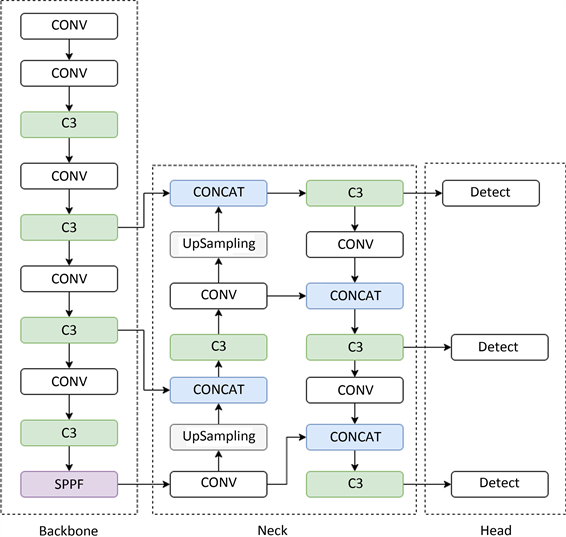Figure 1. YOLOv5s network architectureTable 1. Different anchor box size

2.2.2. 引入特征融合结构Bi-FPN

$P=\underset{i}{\sum }\frac{{w}_{i}}{\epsilon +\sum {\omega }_{j}}\cdot {p}_{i}$ (1)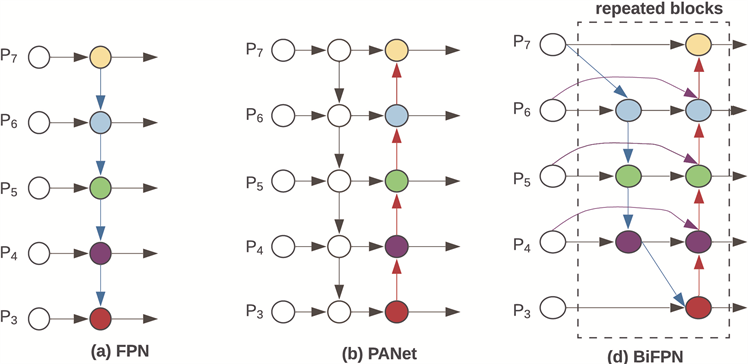Figure 2. FPN and Bi-FPN architecture comparison

2.2.3. 添加注意力机制(GAM)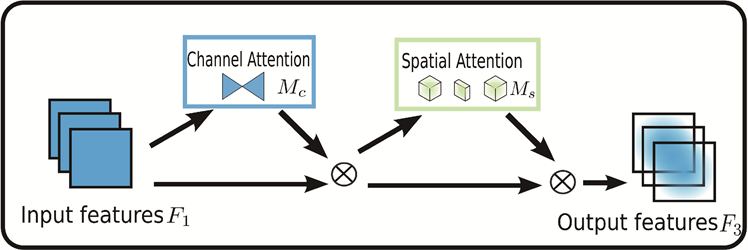Figure 3. The overview of GAM attention

GAM注意力机制(Global Attention Mechanism)  不同于CBAM模块(Convolutional Block Attention Module) ，它不仅仅考虑了将注意力映射沿特征图的通道与空间两个独立的维度进行注入，更考虑了通道与空间的相互作用，引入并放大了跨维度的交互作用，在所有三个维度上捕捉重要特征。GAM attention整个过程如图3所示。

GAM attention包括通道注意力子模块和空间注意力子模块。通道注意力子模块使用三维排列在三个维度上保留信息，用一个两层的MLP (多层感知器)放大跨维通道–空间依赖性。对于输入的特征图，首先进行维度转换，经过维度转换的特征图输入到MLP，再转换为原来的维度，并进行Sigmoid处理输出(见图4)。空间注意力子模块使用两个卷积层进行空间信息融合，删除了池化层对空间位置信息的损失影响，利用通道缩减和7*7的卷积来减少通道数量，在减少计算量的同时专注于对于空间维度信息的提取(见图5)。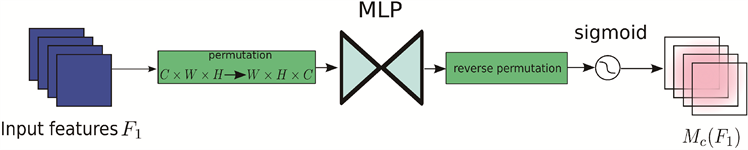Figure 4. Channel attention submodule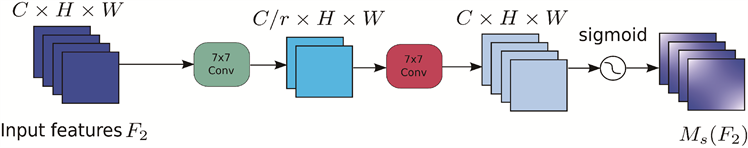Figure 5. Spatial attention submodule

2.2.4. 边界回归损失函数的改进

YOLOv5的损失函数由分类损失(classification loss)、目标置信度损失(object loss)和定位框bbox回归损失(boundingbox regression loss)三部分组成。其中定位框bbox回归损失采用完全交并比损失函数(Complete-IoU loss, CIoU Loss )实现目标框预测。具体计算如公式(2)~(5)所示。

${\mathcal{L}}_{CIoU}=1-CIoU$ (2)

$CIoU=IoU\left(B,{B}^{gt}\right)+\frac{{\rho }^{2}\left(B,{B}^{gt}\right)}{{C}^{2}}+\alpha v$ (3)

$v=\frac{4}{\text{π}}\left(\mathrm{arctan}\frac{{w}^{gt}}{{h}^{gt}}-\mathrm{arctan}\frac{w}{n}\right)$ (4)

$\alpha =\frac{v}{1-IoU\left(B,{B}^{gt}\right)+v}$ (5)

SIoU考虑了真实框和预测框之间的向量角度，重新定义了惩罚指标。具体包含四个部分：角度损失(Angle cost)、距离损失(Distance cost)、形状损失(Shape cost)、IoU损失(IoU cost)。

a) 角度损失

$\Lambda =1-2\ast {\mathrm{sin}}^{2}\left(\mathrm{arcsin}x-\frac{\pi }{4}\right)$ (6)

$x=\frac{{C}_{h}}{\sigma }=\mathrm{sin}\left(\alpha \right)$ (7)

$\sigma =\sqrt{{\left({b}_{{c}_{x}}^{gt}-{b}_{{c}_{x}}\right)}^{2}+{\left({b}_{{c}_{y}}^{gt}-{b}_{{c}_{y}}\right)}^{2}}$ (8)

${c}_{h}=\text{max}\left({b}_{{c}_{y}}^{gt},{b}_{{c}_{y}}\right)-\text{min}\left({b}_{{c}_{y}}^{gt},{b}_{{c}_{y}}\right)$ (9)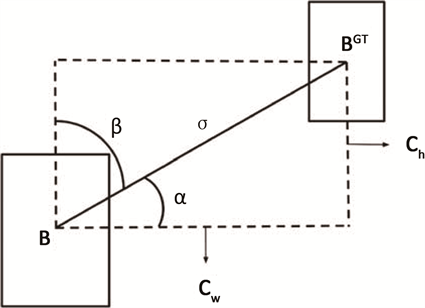Figure 6. Angle cost

b) 距离损失

$\Delta ={\Sigma }_{t=x,y}\left(1-{\text{e}}^{-r\rho t}\right)$ (10)

$\rho =\frac{{\left({b}_{{c}_{x}}^{gt}-{b}_{{c}_{x}}\right)}^{2}}{{c}_{w}},\text{\hspace{0.17em}}\rho =\frac{{\left({b}_{{c}_{y}}^{gt}-{b}_{{c}_{y}}\right)}^{2}}{{c}_{h}},\text{\hspace{0.17em}}r=2-\Lambda$ (11)

c) 形状损失

$\Omega =\underset{t=w,h}{\sum }{\left(1-{\text{e}}^{-{\omega }_{t}}\right)}^{\theta }$ (12)

${\omega }_{w}=\frac{|w-{w}^{gt}|}{\mathrm{max}\left(w,{w}^{gt}\right)}$ (13)

${\omega }_{h}=\frac{|h-{h}^{gt}|}{\mathrm{max}\left(h,{h}^{gt}\right)}$ (14)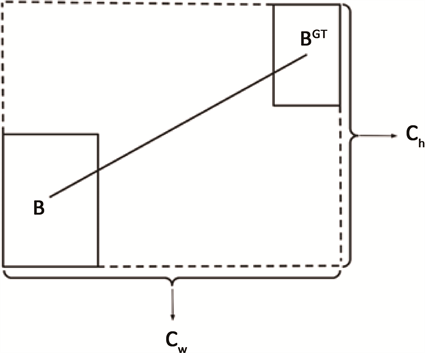Figure 7. Shape cost

${\mathcal{L}}_{SIoU}=1-\text{IoU}\left(B,{B}^{gt}\right)+\frac{\Omega +\Delta }{2}$ (15)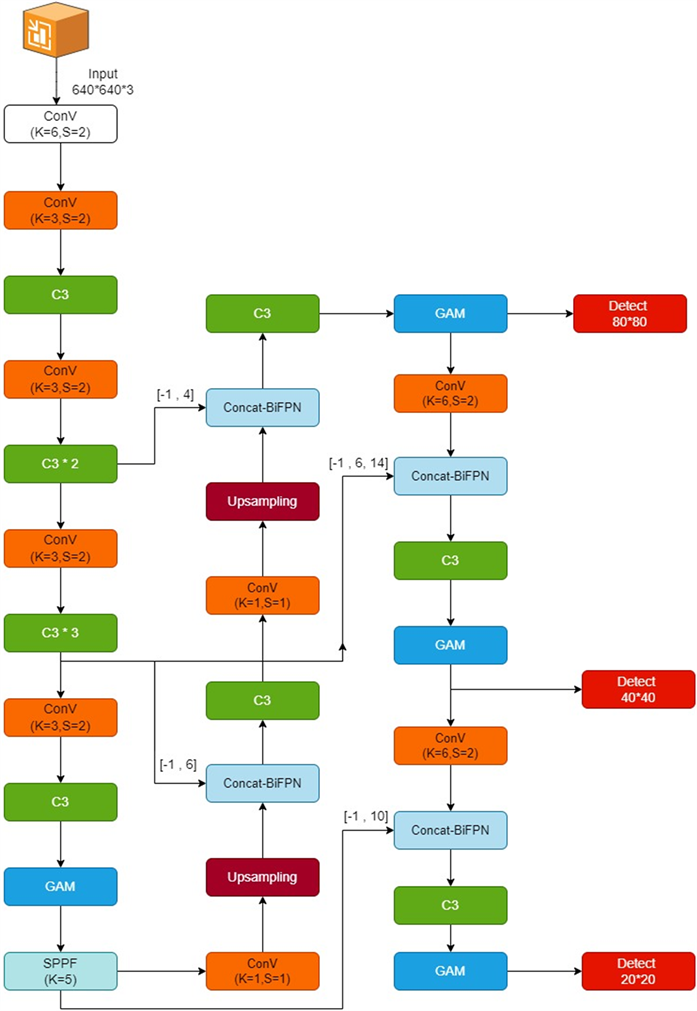Figure 8. Improved YOLOv5 network architecture

3. 实验结果与分析

3.1. 数据集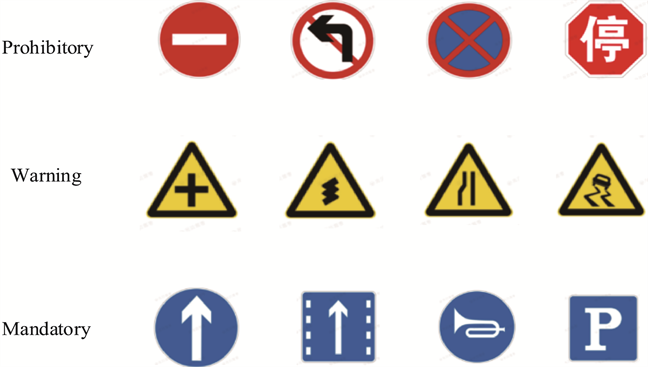Figure 9. Chinese traffic signs with meaningful classificationTable 2. Traffic sign dataset classification and amountTable 3. Cropped image size and amounts

3.2. 实验环境和设定细节

3.3. 评价标准

$P=\frac{TP}{TP+FP}$

$R=\frac{TP}{TP+FN}$

${F}_{1}=\frac{2PR}{P+R}$

$mAP=\frac{1}{N}\underset{i=1}{\overset{N}{\sum }}\underset{0}{\overset{1}{\int }}P\left(R\right)\text{d}R$

3.4. 实验结果及分析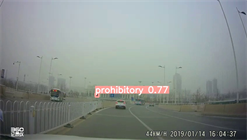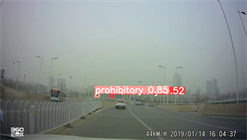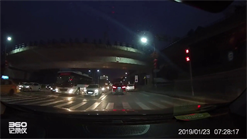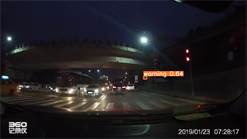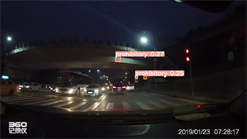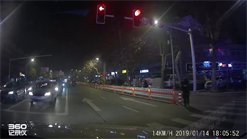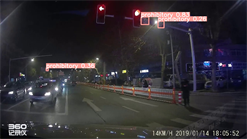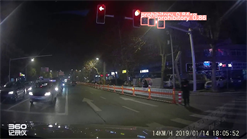(a) 原图(b) YOLOV5s算法 (c) 改进的YOLOv5s算法

Figure 10. Improved YOLOv5 detection result comparisonTable 5. Experiments result for classificationTable 6. Experiments results for cropped image size

4. 结论

  孙颖, 葛平淑, 刘德全. 交通标志的检测与识别研究综述[J]. 大连民族大学学报, 2019, 21(5): 412-417.  陈飞, 刘云鹏, 李思远. 复杂环境下的交通标志检测与识别方法综述[J]. 计算机工程与应用, 2021, 57(16): 65-73.  伍晓晖, 田启川. 交通标志识别方法综述[J]. 计算机工程与应用, 2020, 56(10): 20-26.  马健, 张敏, 张丽岩, 段晓科. 交通标志识别系统研究综述[J]. 物流科技, 2021, 44(10): 69-74. https://doi.org/10.13714/j.cnki.1002-3100.2021.10.022  Girshick, R., Donahue, J., Darrell, T. and Malik, J. (2014) Rich Feature Hierarchies for Accurate Object Detection and Semantic Segmentation. 2014 IEEE Confer-ence on Computer Vision and Pattern Recognition, Columbus, 23-28 June 2014, 580-587. https://doi.org/10.1109/CVPR.2014.81  Girshick, R. (2015) Fast R-CNN. 2015 IEEE International Conference on Computer Vision, Santiago, 7-13 December 2015, 1440-1448. https://doi.org/10.1109/ICCV.2015.169  Ren, S., He, K., Girshick, R. and Sun, J. (2015) Faster R-CNN: Towards Real-Time Object Detection with Region Proposal Networks. IEEE Transactions on Pattern Analysis and Machine Intelligence, 39, 1137-1149. https://doi.org/10.1109/TPAMI.2016.2577031  Liu, W., Anguelov, D., Erhan, D., et al. (2016) SSD: Single Shot Multibox Detector. In: Leibe, B., Matas, J., Sebe, N. and Welling, M., Eds., Computer Vision—ECCV 2016. Lecture Notes in Computer Science, Springer, Cham, 21-37. https://doi.org/10.1007/978-3-319-46448-0_2  Redmon, J., Divvala, S., Girshick, R. and Farhadi, A. (2016) You Only Look Once: Unified, Real-Time Object Detection. 2016 IEEE Conference on Computer Vision and Pattern Recognition, Las Vegas, 27-30 June 2016, 779-788. https://doi.org/10.1109/CVPR.2016.91  Redmon, J. and Farhadi, A. (2017) YOLO9000: Better, Faster, Stronger. 2017 IEEE Conference on Computer Vision and Pattern Recognition, Honolulu, 21-26 July 2017, 6517-6525. https://doi.org/10.1109/CVPR.2017.690  Redmon, J. and Farhadi, A. (2018) Yolov3: An Incremental Improvement. ArXiv: 1804.02767.  Bochkovskiy, A., Wang, C.Y. and Liao, H.Y.M. (2020) Yolov4: Optimal Speed and Accuracy of Object Detection. ArXiv: 2004.10934.  李旭东, 张建明, 谢志鹏, 王进. 基于三尺度嵌套残差结构的交通标志快速检测算法[J]. 计算机研究与发展, 2020, 57(5): 1022-1036.  鲍敬源, 薛榕刚. 基于YOLOv3模型压缩的交通标志实时检测算法[J]. 计算机工程与应用, 2020, 56(23): 202-210.  刘万军, 李嘉欣, 曲海成. 基于多尺度卷积神经网络的交通标示识别研究[J]. 计算机应用研究, 2022, 39(5): 1557-1562. https://doi.org/10.19734/j.issn.1001-3695.2021.10.0457  Zhang, J., Zou, X., Kuang, L.-D., Wang, J., Sherratt, R.S. and Yu, X. (2022) CCTSDB 2021: A More Comprehensive Traffic Sign Detection Benchmark. Human-Centric Computing and Information Sciences, 12, Article No. 23. https://doi.org/10.22967/HCIS.2022.12.023  Wang, C.Y., Liao, H.Y.M., Wu, Y.H., et al. (2020) CSPNet: A New Backbone That Can Enhance Learning Capability of CNN. 2020 IEEE/CVF Conference on Computer Vision and Pattern Recognition Workshops, Seattle, 14-19 June 2020, 390-391. https://doi.org/10.1109/CVPRW50498.2020.00203  He, K., Zhang, X., Ren, S. and Sun, J. (2015) Spatial Pyramid Pooling in Deep Convolutional Networks for Visual Recognition. IEEE Transactions on Pattern Analysis and Machine Intelligence, 37, 1904-1916. https://doi.org/10.1109/TPAMI.2015.2389824  Lin, T.Y., Dollár, P., Girshick, R., et al. (2017) Feature Pyramid Networks for Object Detection. 2017 IEEE Conference on Computer Vision and Pattern Recognition, Honolulu, 21-26 July 2017, 936-944. https://doi.org/10.1109/CVPR.2017.106  Liu, S., Qi, L., Qin, H., et al. (2018) Path Aggregation Network for Instance Segmentation. 2018 IEEE Conference on Computer Vision and Pattern Recognition, Salt Lake City, 18-23 June 2018, 8759-8768. https://doi.org/10.1109/CVPR.2018.00913  Tan, M., Pang, R. and Le, Q.V. (2020) Efficientdet: Scalable and Efficient Object Detection. 2020 IEEE/CVF Conference on Computer Vision and Pattern Recognition, Seattle, 13-19 June 2020, 10778-10787. https://doi.org/10.1109/CVPR42600.2020.01079  Liu, Y., Shao, Z. and Hoffmann, N. (2021) Global At-tention Mechanism: Retain Information to Enhance Channel-Spatial Interactions. ArXiv: 2112.05561.  Woo, S., Park, J., Lee, J.Y. and Kweon, I.S. (2018) CBAM: Convolutional Block Attention Module. Proceedings of the Eu-ropean Conference on Computer Vision (ECCV), Cham, 3-19. https://doi.org/10.1007/978-3-030-01234-2_1  Zheng, Z., Wang, P., Ren, D., et al. (2021) Enhancing Geometric Factors in Model Learning and Inference for Object Detection and Instance Segmentation. IEEE Trans-actions on Cybernetics, 52, 8574-8586. https://doi.org/10.1109/TCYB.2021.3095305  Rezatofighi, H., Tsoi, N., Gwak, J.Y., et al. (2019) Gener-alized Intersection over Union: A Metric and a Loss for Bounding Box Regression. 2019 IEEE/CVF Conference on Computer Vision and Pattern Recognition, Long Beach, 15-20 June 2019, 658-666. https://doi.org/10.1109/TCYB.2021.3095305  Gevorgyan, Z. (2022) SIoU Loss: More Powerful Learning for Bounding Box Regression. ArXiv: 2205.12740.